## Program Verification with F*

### Danel Ahman

#### University of Ljubljana

Tallinn, 20 November, 2019

### Bio

• 2007 - 2010, Tallinn University of Technology, BSc (virtualisation)

• 2011 - 2012, University of Cambridge, MPhil (computational effects)

• 2012 - 2017, University of Edinburgh, PhD (effects & dependent types)

• 2011, Institute of Cybernetics, Research Intern (container datatypes)

• 2014, Microsoft Research Silicon Valley, Research Intern (big data)

• 2016, Microsoft Research Redmond, Research Intern (F*)

• 2017 - 2018, Inria Paris, PostDoc (F*)

• 2018 - 2019 , University of Ljubljana, PostDoc (effects & F*)

• 2019 - . . . , University of Ljubljana, Marie Curie Fellow (effects & F*)

### Today's plan

• Specification and Verification of Programs

• What is F*?

• Verifying Purely Functional Programs in F*

• Verifying Stateful Programs in F*

• Highlights of Other F* Features

Slides, code, exercises, homework, and setup instructions
https://danel.ahman.ee/teaching/taltech2019/

## Specification and Verification

### Program specification

• Consider a simple purely functional list reversal
let rec rev #a (l:list a) : list a =
match l with
| []     -> []
| hd::tl -> append (rev tl) [hd]
• The specification of rev could comprise a variety of properties, e.g.,

1. rev (rev l) == l
2. length (rev l) == length l
3. rev l  contains the same elements as  l
4. if  sorted (>=) l  then  sorted (<=) (rev l)
• Formally, specifications are often written as Hoare triples
{requires (sorted (>=) l)}                   |        {requires (True)}
rev l                                      |          rev (rev l)
{ensures (fun l' -> sorted (<=) l')}         |        {ensures (fun l' -> l == l')}

### Program specification ctd.

• Now consider a simple stateful list reversal
let rec srev #a (l:ref (list a)) : unit =
match !l with
| []     -> ()
| hd::tl -> l := tl; srev tl; l := (append !l [hd])
• The specification of srev could comprise a variety of properties, e.g.,

• previous functional properties   +   memory safety
• Formally, specifications are still written as Hoare triples
{requires (fun h0 -> sorted (>=) (sel h0 l))}
srev l
{ensures (fun h0 _ h1 -> sorted (<=) (sel h1 l) /\ modifies !{l} h0 h1)}
• What about other effects such as exceptions, input-output, ?

### Program verification

#### Verifying a program is to show that itsatisfies its specification, e.g.,

• Code reviews
• Testing (unit testing, randomised testing, model-based testing, )
• Runtime verification (monitors, )
• Program logics (Hoare logic, separation logic, )

{fun h0 -> sorted (>=) (sel h0 l)} srev l {fun h0 _ h1 -> sorted (<=) (sel h1 l)}

{fun h1 -> sorted (<=) (sel h1 l)} srev l {fun h1 _ h2 -> sorted (>=) (sel h2 l)}
---------------------------------------------------------------------------------
{fun h0 -> sorted (>=) (sel h0 l)}
srev l; srev l
{fun h1 _ h2 -> sorted (>=) (sel h2 l)}
• Expressive type systems (dependent types, refinement types, )

rev : l:list a -> (l':list a & (length l == length l'))

## What is F*?

### Program verification: Shall the twain ever meet?

Interactive proof assistants Semi-automated verifiers of
imperative programs
Coq,   air         Dafny,
Agda,       FramaC,
Lean,   gap         Why3,
• Left side: very expressive logics, interactive proving, tactics

• but mostly only purely functional programming

• Right side: effectful programming, SMT-based automation

• but only very weak logics

### Bridging the air gap: F*

• Functional programming language with effects

let incr = fun (r:ref a) -> r := !r + 1

but with a much richer type system

• by default extracted to OCaml or F#

• subset extracted to efficient C code (Low* and KreMLin)

• Semi-automated verification system using SMT (Z3)
• push-button automation like in Dafny, FramaC, Why3, Liquid Haskell,
• Interactive proof assistant based on dependent types
• interactive proving and tactics like in Coq, Agda, Lean,

### F* in action, at scale

• Functional programming language with effects

• F* is programmed in F*, but not (yet) verified
• Semi-automated verification system

• Project Everest: verify and deploy new, efficient HTTPS stack
• miTLS: Verified reference implementation of TLS
• HACL*: High-Assurance Crypto Library (used in Firefox, Wireguard VPN, Zinc kernel crypto library, Tezos and Concordium blockchains)
• Vale: Verified Assembly Language for Everest
• Proof assistant based on dependent types

• Fallback when SMT fails; also for mechanized metatheory
• Wys*: Verified DSL for secure multi-party computations
• ReVerC: Verified compiler to reversible circuits
• Meta-F* (metaprogramming and tactics) increasingly used in Everest

## The current F* team

Microsoft Research (US, UK, India), Inria Paris, MIT, Rosario, …

Danel Ahman
Benjamin Beurdouche
Karthikeyan Bhargavan
Barry Bond
Antoine Delignat-Lavaud
Victor Dumitrescu
Cédric Fournet
Chris Hawblitzel
Cătălin Hriţcu
Markulf Kohlweiss
Qunyan Mangus
Kenji Maillard
Asher Manning
Guido Martínez
Clément Pit-Claudel
Jonathan Protzenko
Ramkumar Ramachandra
Tahina Ramananandro
Aseem Rastogi
Nikhil Swamy (benevolent dictator)
Christoph M. Wintersteiger
Santiago Zanella-Béguelin
Gustavo Varo

### How to use F*

• Two kinds of F* files

• A.fsti - interface file for module called A (can be omitted)

• A.fst - source code file for module called A

• Command line: typechecking/verification
  $fstar.exe Ackermann.fst Verified module: Ackermann (429 milliseconds) All verification conditions discharged successfully • Command line: typechecking/verification + program extraction $ fstar.exe Ackermann.fst --odir out-dir --codegen OCaml
• Interactive: development + verification (Emacs with fstar-mode)

### The functional core of F*

• Recursive functions

val factorial : nat -> nat

let rec factorial n =
if n = 0 then 1 else n * (factorial (n - 1))
• (Simple) inductive datatypes and pattern matching

type list (a:Type) =
| Nil  : list a
| Cons : hd:a -> tl:list a -> list a

let rec map (f:'a -> 'b) (x:list 'a) : list 'b =
match x with
| Nil      -> Nil
| Cons h t -> Cons (f h) (map f t)
• Lambdas

map (fun x -> x + 42) [1;2;3]

### Refinement types

type nat = x:int{x >= 0}                      (* general form x:t{phi x} *)
• Refinements introduced by type annotations (code unchanged)

val factorial : nat -> nat
let rec factorial n = if n = 0 then 1 else n * (factorial (n - 1))
• Logical obligations discharged by SMT (for else branch, simplified)

n >= 0, n <> 0 |= n - 1 >= 0
n >= 0, n <> 0, (factorial (n - 1)) >= 0 |= n * (factorial (n - 1)) >= 0
• Refinements eliminated by subtyping:  nat <: int

let i : int = factorial 42         let f : x:nat{x > 0} -> int = factorial
• Refinement formulae built from standard logical connectives

•  == ,  =!= ,  /\ ,  \/ ,  ~ ,  forall ,  exists  ,  ...

### Dependent types

• Dependent function types aka -types

val incr : x:int -> y:int{x < y}
let incr x = x + 1
• Can express pre- and postconditions of pure functions

val incr' : x:nat{odd x} -> y:nat{even y}
• (Parameterised and indexed) inductive datatypes; implicit arguments

type vec (a:Type) : nat -> Type =
| Nil  : vec a 0
| Cons : #n:nat -> hd:a -> tl:vec a n -> vec a (n + 1)

let rec map (#n:nat) (#a #b:Type) (f:a -> b) (as:vec a n) : vec b n =
match as with
| Nil        -> Nil
| Cons hd tl -> Cons (f hd) (map f tl)

### Inductive families + refinement types

• In Coq or Agda, we have to carry around explicit proofs, e.g.,

type vec (a:Type) : nat -> Type =
| Nil  : vec a 0
| Cons : #n:nat -> hd:a -> tl:vec a n -> vec a (n + 1)

let rec lookup #a #n (as:vec a n) (i:nat) (p:i less_than n) : a = ...
• Combining vec with refinement types is much more convenient

let rec lookup #a #n (as:vec a n) (i:nat{i < n}) : a =
match as with
| Cons hd tl -> if i = 0 then hd else lookup tl (i - 1)
• Often even more convenient to use simple lists + refinement types

let rec length #a (as:list a) : nat =
match as with
| []       -> 0
| hd :: tl -> 1 + length tl

let rec lookup #a (as:list a) (i:nat{i < (length as)}) : a =
match as with
| hd :: tl -> if i = 0 then hd else lookup tl (i - 1)

### Total functions in F*

• The F* functions we saw so far were all total

• Tot effect (default) = no side-effects, terminates on all inputs

(* val factorial : nat -> nat *)

val factorial : nat -> Tot nat

let rec factorial n =
if n = 0 then 1 else n * (factorial (n - 1))
• Quiz: How about giving this weaker type to factorial?

val factorial : int -> Tot int
  let rec factorial n = if n = 0 then 1 else n * (factorial (n - 1))
^^^^^
Subtyping check failed; expected type (x:int{(x << n)}); got type int

factorial (-1) loops!    (int type in F* is unbounded)

### The divergence effect (Dv)

• We might not want to prove all code terminating

val factorial : int -> Dv int
• Some useful code really is not always terminating

• evaluator for lambda terms
val eval : exp -> Dv exp
let rec eval e =
match e with
| App (Lam x e1) e2 -> eval (subst x e2 e1)
| App e1 e2         -> eval (App (eval e1) e2)
| Lam x e1          -> Lam x (eval e1)
| _                 -> e

let main () = eval (App (Lam 0 (App (Var 0) (Var 0)))
(Lam 0 (App (Var 0) (Var 0))))
./Divergence.exe
• servers

### Effect encapsulation (Tot and Dv)

• Pure code cannot call potentially divergent code

• Only (!) pure code can appear in specifications

val factorial : int -> Dv int

type tau = x:int{x = factorial (-1)}
type tau = x:int{x = factorial (-1)}
^^^^^^^^^^^^^^^^^^
Expected a pure expression; got an expression ... with effect "DIV"
• Sub-effecting:  Tot t <: Dv t

• So, divergent code can include pure code

incr 2 + factorial (-1) : Dv int

### Tot and Dv are just two effects amongst many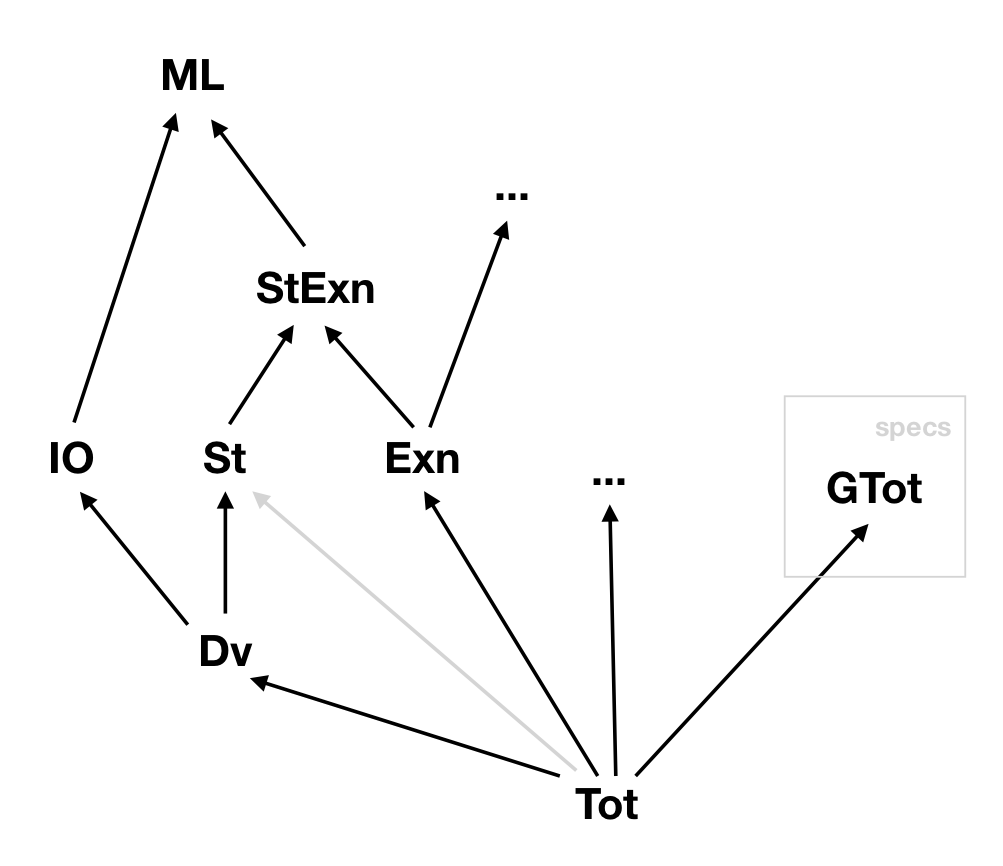### Effect encapsulation (Tot and GTot)

• Ghost effect for code used only in specifications

val sel : #a:Type -> heap -> ref a -> GTot a
• Sub-effecting:  Tot t <: GTot t

• BUT NOT (!):  GTot t <: Tot t  (holds for non-informative types)

• So, (informative) ghost code cannot be used in total functions

let f (g:unit -> GTot nat) : Tot (n:nat{n = g ()}) = g ()
Computed type "n:nat{n = g ()}" and effect "GTot"
is not compatible with the annotated type "n:nat{n = g ()}" effect "Tot"
• But total functions can appear in ghost code (regardless of their type)

let f (g:unit -> Tot nat) : GTot (n:nat{n = g ()}) = g ()

### Verifying pure programs

#### Variant #1:intrinsically (at definition time)

• Using refinement types (saw this already)
val factorial : nat -> Tot nat              (* type nat = x:int{x >= 0} *)
• Can equivalently use pre- and postconditions for this
val factorial : x:int -> Pure int (requires (x >= 0))
(ensures  (fun y -> y >= 0))
• Each F* computation type is of the form
• effect (e.g. Pure)       result type (e.g. int)       spec. (e.g. pre and post)

• Tot is just an abbreviation
Tot t = Pure t (requires True) (ensures (fun _ -> True))

### Verifying pure programs

#### Variant #2:extrinsically using SMT-backed lemmas

let rec append (#a:Type) (xs ys:list a) : Tot (list a) =
match xs with
| []       -> ys
| x :: xs' -> x :: append xs' ys
let rec lemma_append_length (#a:Type) (xs ys:list a)
: Pure unit
(requires True)
(ensures  (fun _ -> length (append xs ys) = length xs + length ys)) =

match xs with
| []       -> ()
(* nil-VC: len (app [] ys) = len [] + len ys                            *)

| x :: xs' -> lemma_append_length xs' ys
(*          len (app xs' ys) = len xs' + len ys                         *)
(* cons-VC:           ==> len (app (x::xs') ys) = len (x::xs') + len ys *)
• Convenient syntactic sugar: the Lemma effect
Lemma property = Pure unit (requires True) (ensures (fun _ -> property))

### Often lemmas are unavoidable

let snoc l h = append l [h]

let rec rev #a (l:list a) : Tot (list a) =
match l with
| []     -> []
| hd::tl -> snoc (rev tl) hd
val lemma_rev_snoc : #a:Type -> l:list a -> h:a ->
Lemma (rev (snoc l h) == h::rev l)

let rec lemma_rev_snoc (#a:Type) l h =
match l with
| []     -> ()
| hd::tl -> lemma_rev_snoc tl h
val lemma_rev_involutive : #a:Type -> l:list a -> Lemma (rev (rev l) == l)

let rec lemma_rev_involutive (#a:Type) l =
match l with
| []     -> ()
| hd::tl -> lemma_rev_involutive tl; lemma_rev_snoc (rev tl) hd

### Often lemmas are unavoidable (but SMT can help)

let snoc l h = append l [h]

let rec rev #a (l:list a) : Tot (list a) =
match l with
| []     -> []
| hd::tl -> snoc (rev tl) hd
val lemma_rev_snoc : #a:Type -> l:list a -> h:a ->
Lemma (rev (snoc l h) == h::rev l)
[SMTPat (rev (snoc l h))]
let rec lemma_rev_snoc (#a:Type) l h =
match l with
| []     -> ()
| hd::tl -> lemma_rev_snoc tl h
val lemma_rev_involutive : #a:Type -> l:list a -> Lemma (rev (rev l) == l)

let rec lemma_rev_involutive (#a:Type) l =
match l with
| []     -> ()
| hd::tl -> lemma_rev_involutive tl (*; lemma_rev_snoc (rev tl) hd*)

### Verifying potentially divergent programs

#### The only variant:intrinsically (partial correctness)

• Using refinement types
val factorial : nat -> Dv nat
• Or the Div computation type (pre- and postconditions)
val eval_closed : e:exp -> Div exp
(requires (closed e))
(ensures  (fun e' -> Lam? e' /\ closed e'))
let rec eval_closed e =
match e with           (* notice there is no match case for variables *)
| App e1 e2 ->
let Lam e1' = eval_closed e1 in
below_subst_beta 0 e1' e2;
eval_closed (subst (sub_beta e2) e1')
| Lam e1 -> Lam e1
• Dv is also just an abbreviation
Dv t = Div t (requires True) (ensures (fun _ -> True))

### Recap: Functional core of F*

• Variant of dependent type theory

• , , inductives, matches, universe polymorphism,
• General recursion and semantic termination check

• potential non-termination is an effect!
• Refinements

• Refined value types:
• x:t{phi x}
• Refined computation types:
• Pure t pre post
• Div t pre post
• refinements computationally and proof irrelevant, discharged by SMT
• Subtyping and sub-effecting (<:)

• Standard logical connectives (==, /\, \/, forall , exists , ...)

## Verifying Stateful Programs in F*

### Verifying stateful programs

• The St effectprogramming with garbage-collected references

val incr : r:ref int -> St unit

let incr r = r := !r + 1
• Hoare logic-style preconditions and postconditions with ST

val incr : r:ref int ->
ST unit (requires (fun h0      -> True))
(ensures  (fun h0 _ h2 -> modifies !{r} h0 h2 /\
sel h2 r == sel h0 r + 1))
• precondition (requires) is a predicate on initial states
• postcondition (ensures) relates initial states, results, and final states
• St is again just an abbreviation

St t = ST t (requires True) (ensures (fun _ -> True))
• Sub-effecting:  Pure <: ST  and  Div <: ST  (partial correctness)

### Heap and ST interfaces (much simplified)

module Heap
val heap : Type
val ref : Type -> Type

val sel : #a:Type -> heap -> ref a -> GTot a
val addr_of : #a:Type -> ref a -> GTot nat
val contains : #a:Type -> heap -> ref a -> Type0

let modifies (s:FStar.TSet.set nat) (h0 h1 : heap) =
forall a (r:ref a) . (~(addr_of r mem s) /\ h0 contains r)
==> sel h1 r == sel h0 r
module ST

val alloc : #a:Type -> init:a ->
ST (ref a) (requires (fun _ -> True))
(ensures  (fun h0 r h1 ->
modifies !{} h0 h1 /\ sel h1 r == init /\ fresh r h0 h1))

val (!) : #a:Type -> r:ref a ->
ST a (requires (fun _       -> True))
(ensures  (fun h0 x h1 -> h0 == h1 /\ x == sel h0 r))

val (:=) : #a:Type -> r:ref a -> v:a ->
ST unit (requires (fun _      -> True))
(ensures  (fun h0 _ h1 -> modifies !{r} h0 h1 /\ sel h1 r == v))

### Verifying incr (intuition)

let incr r = r := !r + 1
val incr : r:ref int ->
ST unit (requires (fun _ -> True))
(ensures  (fun h0 _ h2 -> modifies !{r} h0 h2 /\
sel h2 r == sel h0 r + 1))
val incr : r:ref int ->
ST unit
(requires (fun _ -> True))
(ensures  (fun h0 _ h2 ->
exists h1 x. h0 == h1 /\ x == sel h0 r /\             //(!)
modifies !{r} h1 h2 /\ sel h2 r == x+1)) //(:=)
let incr r =
let x = !r in
r := x + 1
val (!) : #a:Type -> r:ref a ->
ST a (requires (fun _       -> True))
(ensures  (fun h0 x h1 -> h0 == h1 /\ x == sel h0 r))

val (:=) : #a:Type -> r:ref a -> v:a ->
ST unit (requires (fun _       -> True))
(ensures  (fun h0 _ h1 -> modifies !{r} h0 h1 /\ sel h1 r == v))

### Typing rule for let / sequencing (intuition)

val incr : r:ref int ->
ST unit
(requires (fun _ -> True))
(ensures  (fun h0 _ h2 ->
exists h1 x. h0 == h1 /\ x == sel h0 r /\             //(!)
modifies !{r} h1 h2 /\ sel h2 r == x+1)) //(:=)
let incr r =
let x = !r in
r := x + 1

G |- e1 : ST t1 (requires (fun h0 -> pre))
(ensures  (fun h0 x1 h1 -> post))

G, x1:t1 |- e2 : ST t2 (requires (fun h1 -> exists h0 . post))
(ensures  (fun h1 x2 h2 -> post'))
---------------------------------------------------------------------------
G |- let x1 = e1 in e2 : ST t2 (requires (fun h0 -> pre))
(ensures  (fun h x2 h2 ->
exists x1 h1 . post /\ post'))

### Reference swapping (hand proof sketch)

val swap : r1:ref int -> r2:ref int ->
ST unit (requires (fun _       -> True))
(ensures  (fun h0 _ h3 -> modifies !{r1,r2} h0 h3 /\
sel h3 r2 == sel h0 r1 /\
sel h3 r1 == sel h0 r2))
let swap r1 r2 =
let t = !r1 in (* Know (P1) *)
r1 := !r2;     (* Know (P2) *)
r2 := t        (* Know (P3) *)
(* (P1): exists h1 t. h0 == h1 /\ t == sel h0 r1 *)
(* (P2): exists h2. modifies !{r1} h1 h2 /\ sel h2 r1 == sel h1 r2 *)
(* (P3): modifies !{r2} h2 h3 /\ sel h3 r2 == t *)

(* modifies !{r1,r2} h0 h3 follows directly from transitivity of modifies *)

(* sel h3 r2 == sel h0 r1 follows immediately from (P1) and (P3) *)

(* Still to show: sel h3 r1 == sel h0 r2
From (P2) we know that  sel h2 r1 == sel h1 r2 (A)

From (P1) we know that  h0 == h1
which directly gives us  sel h1 r2 == sel h0 r2 (B)

From (P3) we know that  modifies !{r2} h2 h3
which by definition gives us  sel h2 r1 == sel h3 r1 (C)

We conclude by transitivity from (A)+(B)+(C) *)

### Integer reference swapping (the funny way)

val swap_add_sub : r1:ref int -> r2:ref int ->
ST unit (requires (fun _ -> addr_of r1 <> addr_of r2 ))
(ensures  (fun h0 _ h1 -> modifies !{r1,r2} h0 h1 /\
sel h1 r1 == sel h0 r2 /\
sel h1 r2 == sel h0 r1))
r1 := !r1 + !r2;
r2 := !r1 - !r2;
r1 := !r1 - !r2
• Correctness of this variant relies on r1 and r2 not being aliased

• and on int being unbounded (mathematical) integers

### But you don't escape having to come up with invariants

#### Stateful Counting: 1+ 1+ 1+ 1+ 1+ 1+ …

let rec count_st_aux (r:ref nat) (n:nat)
: ST unit (requires (fun _       -> True))
(ensures  (fun h0 _ h1 -> modifies !{r} h0 h1 /\
(* to ensure !{} in count_st *)
sel h1 r == sel h0 r + n
(* sel h1 r == n would be wrong *))) =
if n > 0 then (r := !r + 1;
count_st_aux r (n - 1))
let rec count_st (n:nat)
: ST nat (requires (fun _       -> True))
(ensures  (fun h0 x h1 -> modifies !{} h0 h1 /\
x == n)) =
let r = alloc 0 in
count_st_aux r n;
!r
• You'll see much more involved invariants in the lab exercises

### Summary: Verifying Stateful Programs

• ML-style garbage-collected references

val heap : Type
val ref  : Type -> Type

val sel     : #a:Type -> heap -> ref a -> GTot a
val addr_of : #a:Type -> ref a -> GTot nat

val modifies : s:set nat -> h0:heap -> h1:heap -> Type0
• St effect for simple ML-style programming

let incr (r:ref int) : St unit = r := !r + 1
• ST effect for pre- and postcondition based (intrinsic) reasoning

ST unit (requires (fun h0      -> True))
(ensures  (fun h0 _ h2 -> modifies !{r} h0 h2 /\ sel h2 r == n))
• But that's not all there is to F*'s memory models!

• monotonicity, regions, heaps-and-stacks, resources and sep. logic

### F*'s Memory Models are Based on Monotonicity

val recall_contains #a (r:ref a)
: ST unit (requires (fun _       -> True))
(ensures  (fun h0 _ h1 -> h0 == h1 /\
h1 contains r))
• val (!) : #a:Type -> r:ref a ->
ST a (requires (fun _       -> True))
(ensures  (fun h0 x h1 -> h0 == h1 /\
x == sel h0 r))

val (:=) : #a:Type -> r:ref a -> v:a ->
ST unit (requires (fun _      -> True))
(ensures  (fun h0 _ h1 -> modifies !{r} h0 h1 /\
sel h1 r == v))
• Moreover,  ref a  is actually a  mref a rel  with a trivial  rel

val mref : a:Type -> rel:preorder a -> Type

let ref a = mref a (fun _ _ -> True)

### Monotonic References  mref a rel

• Such monotonic references also come with a modal operator

val token #a #rel (r:mref a rel) : (a -> Type0) -> Type0
• And corresponding introduction and elimination rules (stateful progs.)

val witness_token #a #rel (r:mref a rel) (p:(a -> Type0))

: ST unit (requires (fun h0      -> p (sel h0 r) /\ stable p rel))
(ensures  (fun h0 _ h1 -> h0 == h1 /\ token r p))

val recall_token #a #rel (r:mref a rel) (p:(a -> Type0))

: ST unit (requires (fun _       -> token r p))
(ensures  (fun h0 _ h1 -> h0 == h1 /\ p (sel h1 r)))
• Enabling the following useful verification pattern

  let p (x:nat) = x > 0 in                       (* assuming (r : mref nat <=) *)

r := !r + 1;  witness r p;  black_box r;  recall r p;  assert (p !r)
• Examples: counters, logs, network traffic history, state continuity,

## Highlights of Other F* Features

### Low*: verifying low-level C code

#### Moto: The code (Low*) is low-level but the verification (F*) is not

let f (): Stack UInt64.t (requires (fun h0      -> True))
(ensures  (fun h0 r h1 -> r = 43UL))

= push_frame ();                         (* pushing a new stack frame *)

let b = LowStar.Buffer.alloca 1UL 64ul in
assert (b.(42ul) = 1UL);      (* high-level reasoning in F*'s logic *)

b.(42ul) <- b.(42ul) +^ 42UL;
let r = b.(42ul) in

pop_frame ();           (* popping the stack frame we pushed above, *)
(* necessary for establishing Stack invariant *)
r
uint64_t f()
{
uint64_t b[64U];

for (uint32_t _i = 0U; _i < (uint32_t)64U; ++_i)
b[_i] = (uint64_t)1U;

b[42U] = b[42U] + (uint64_t)42U;
uint64_t r = b[42U];
return r;
}

### F* has an extensible effect system

• In addition to Tot, St, , users can define their own (monadic) effects

• Axiomatically (PLDI 2013)

• Only signatures of effect operations.
• Implementation in code extraction.

• User provides a monadic effect definition
• F* then derives the effect and the specification calculus
• Dijkstra Monads For All (ICFP 2019)

• User defines both the computational and specification monads.
• Comp. and spec. monads are related by effect observations.
• Layered Effects (landed in master just a few weeks ago)

• New effects on top of existing ones (like with monads in Haskell).

### Meta-F* - a tactics and metaprogramming framework for F*

• Tactics are just another F* effect (proof state + exceptions)

• Can access the proof state, can introspect and synthesise F* terms

• Run using the normalizer (slow) or compiled to native OCaml plugins

• Uses: discharging VCs, massaging VCs, synthesizing terms, typeclasses

### Tactics can discharge verification conditions (replacing SMT)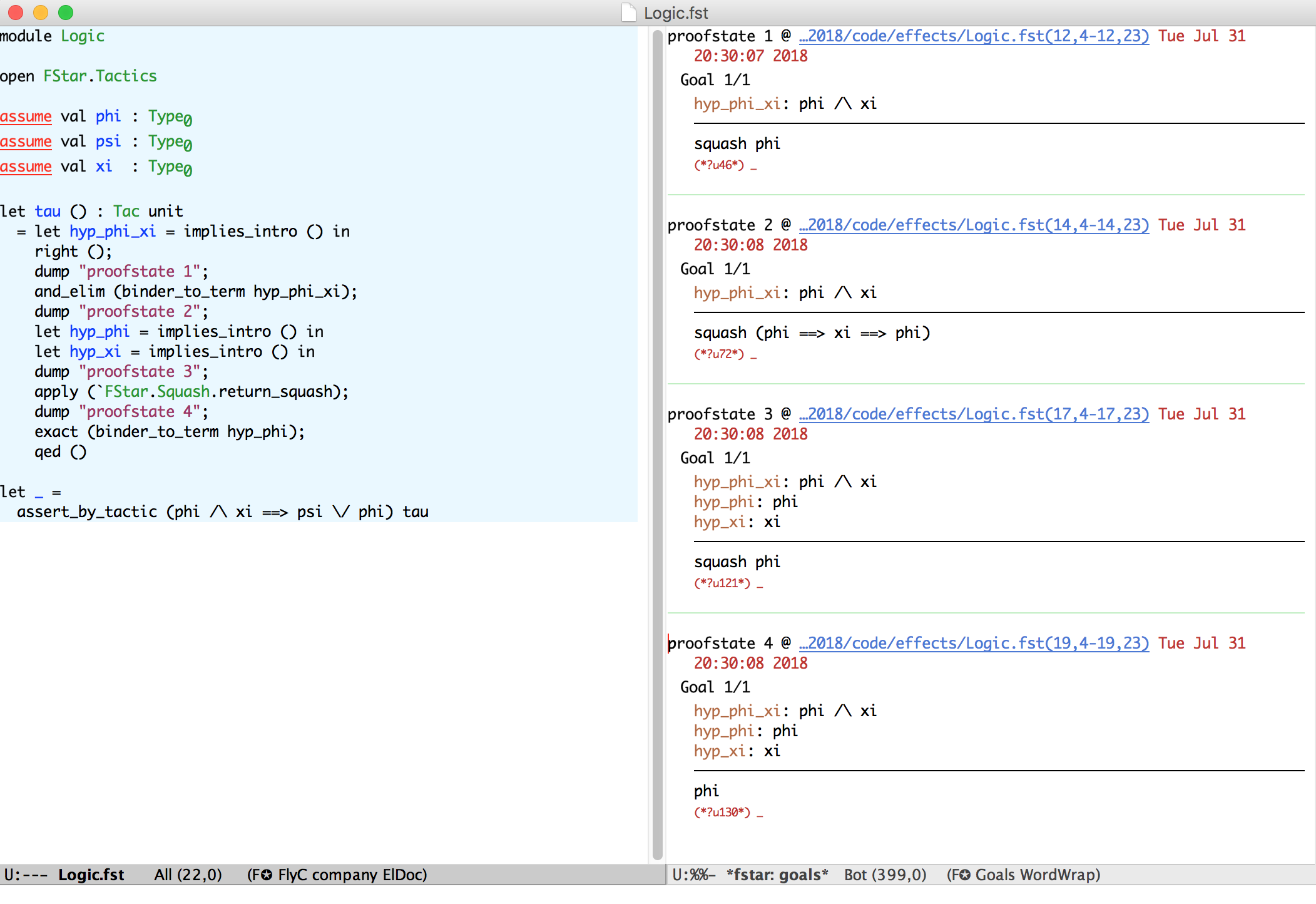### Tactics can massage verification conditions (complementing SMT)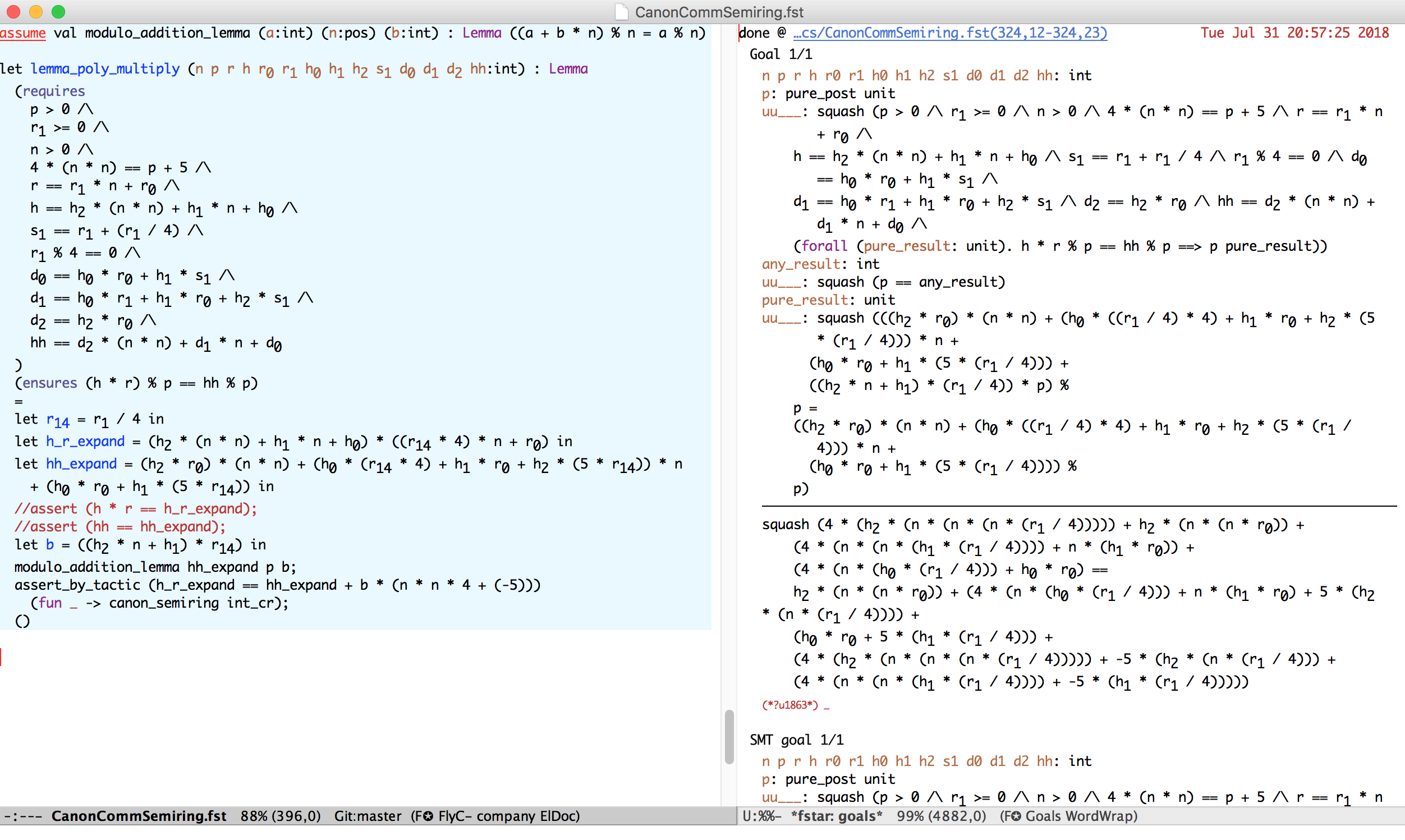### Tactics can synthesize F* terms (metaprogramming)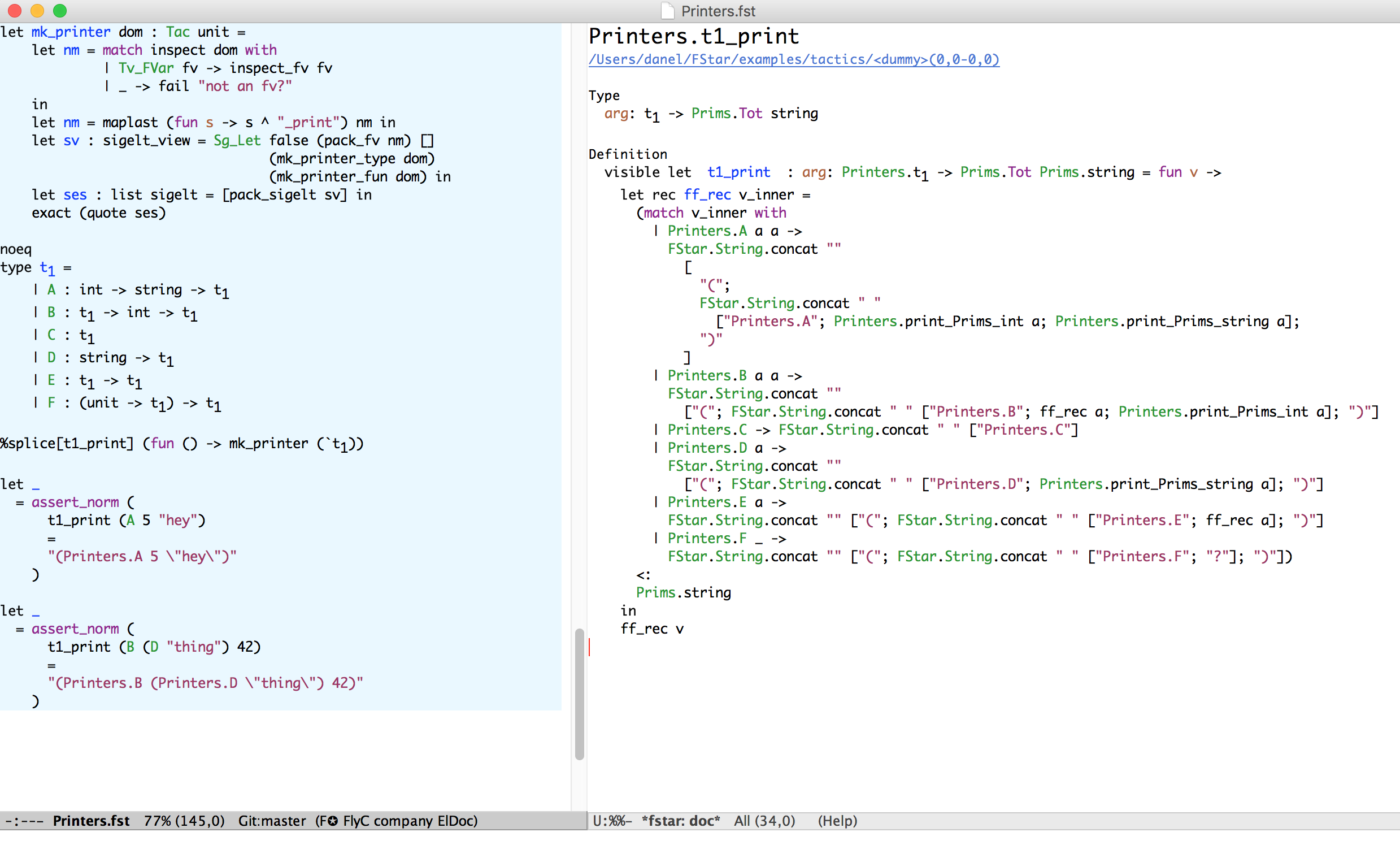### Tactics have also been used to extend F* with typeclasses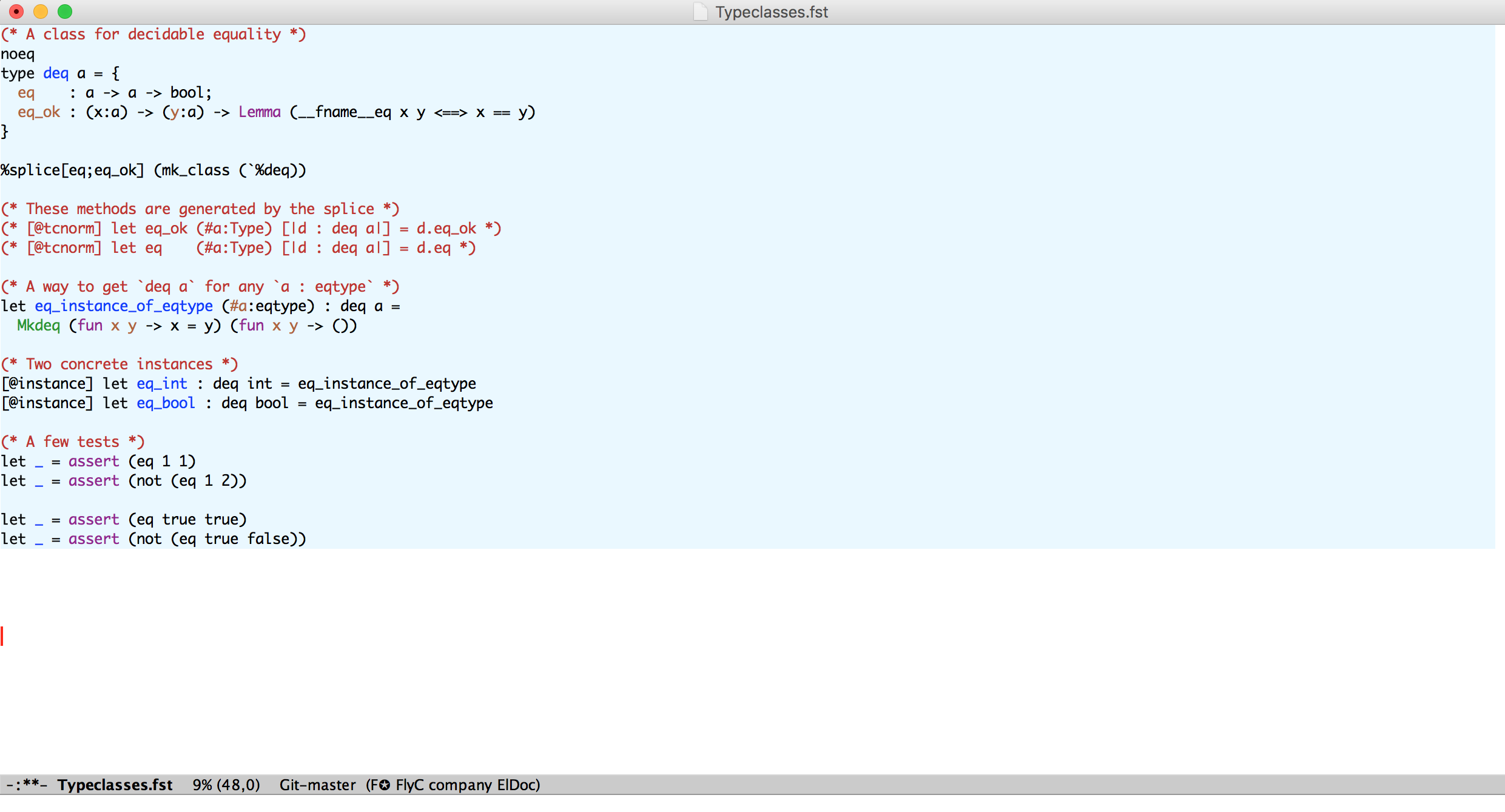### F*

• An ML-style effectful functional programming language

• A semi-automated SMT-based program verifier

• An interactive dependently typed proof assistant

• Used successfully in security and crypto verification

• miTLS: F*-verified reference implementation of TLS

• HACL*: F*-verified crypto (used in Firefox and Wireguard)

• Vale: F*-verified assembly language

• Small exercises for the lab sessions to try out F* yourselves

• verifying pure and stateful programs, using monotonicity,